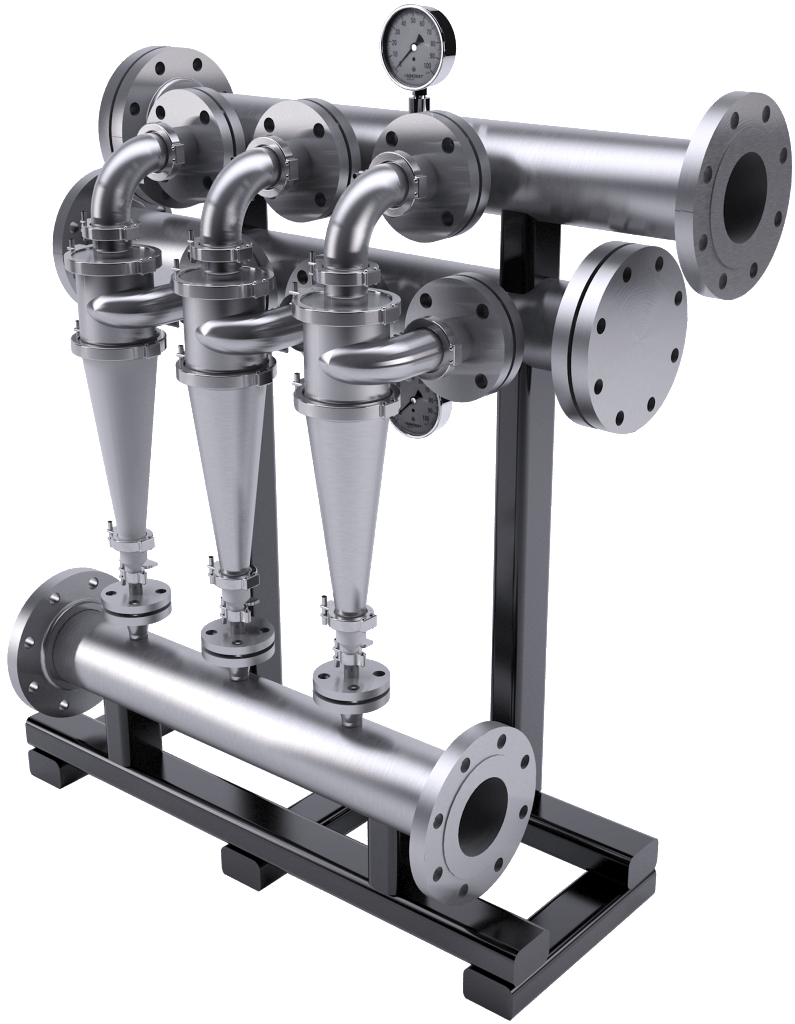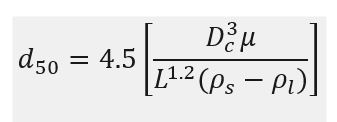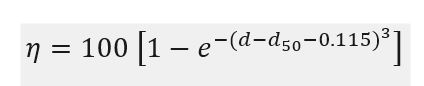# Preliminary Sizing of Hydrocyclones

In this post, I want to share how to do preliminary sizing of hydrocyclones. In previous post, hydrocyclones are utilized for solid-liquid separations. The centrifugal force is produced by the motion of the liquid in this centrifugal device, which has a stationary wall. Like a gas cyclone, the gas cyclone operates on much the same principles. Hydrocyclones are inexpensive, reliable separators that work with particle sizes ranging from 4 to 500 micron. Figure below shows hydrocyclones typical proportion geometry.

Preliminary sizing of hydrocyclones can be done by using nomograph methods. The optimal arrangements and design for a certain application should be determined by consulting the expert manufacturers of hydrocyclone equipment.

Empirical equation below is used to estimate diameter of cyclone chamber.Where:

d50      = the particle diameter for which the cyclone is 50% efficient, μm

Dc       = diameter of cyclone chamber, cm

μ        = liquid viscosity, cP

L         = feed flow rate, l/min

ρL       = density of liquid, g/cm3

ρg       = density of gas, g/cm3

Equation above determines the hydrocyclone chamber diameter necessary to separate the so-called d50 particle diameter as a function of the slurry flow velocity and the physical characteristics of the liquid and solid. The separating efficiency for other particles is related to the d50 diameter which is based on a formula:Where:

ƞ        = the efficiency of the cyclone in separating any particle of diameter d, in percent

d        = the selected particle diameter, μm

The use of nomograph will be presented by using example below.

Example

Estimate the size of hydrocyclone needed to separate 90% of particles with a diameter greater than 20 μm, from 10 m3/h of a dilute slurry.

Physical properties:

Solid density 2000 kg/m3

Liquid density 1000 kg/m3

Viscosity 1 cP

Solution

Flow rate = 10 m3/h = 166.7 liter/min

ρS – ρL = 2 – 1 = 1 g/cm3

Based on figure below, for 90% removal of particles above 20 μm,

d50 = 14 μm